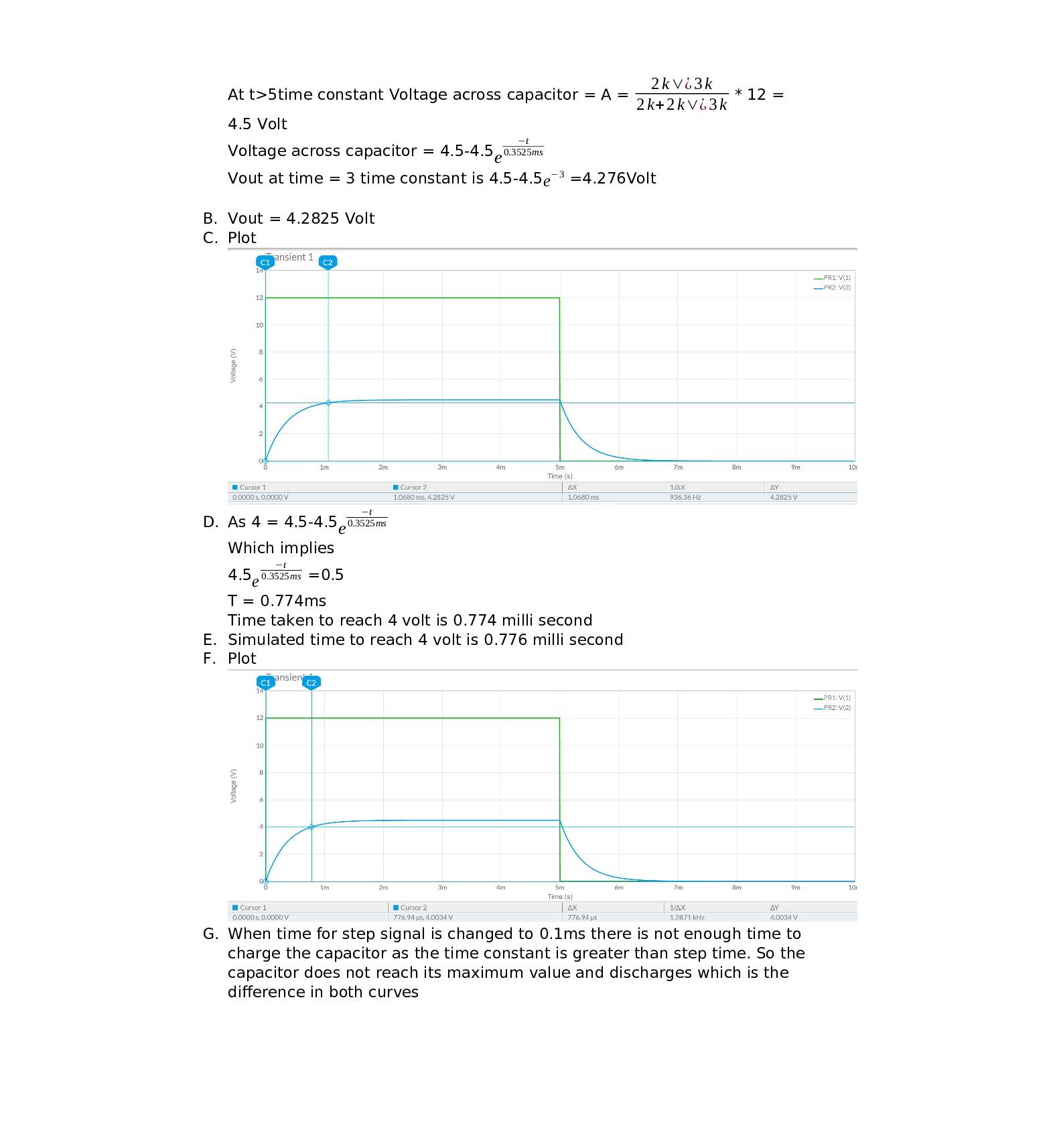Question

Circuits

For the circuit above where VIN is shown here :

a. Calculate the value of VOUT after 3 Time Constants from To.

b. Simulate VIn and Vout then, using cursors, show the value of VouT after 3 TimeConstants from To. Simulated Vout (3 Time Constants) =

c. Copy a picture of simulated VIn and Vout with cursors showing answers from part b.

d. Calculate the time for VouT to reach 4 Volts from To.Calculated time for Vour to reach 4 Volts =

e. Simulate VInand VouT, then using cursors, show the time for Vout to reach 4 Volts .Simulated time for Vout to reach 4 Volts =

f. Copy a picture of simulated VIN and VOUT with cursors showing answers from part e.

Explain qualitatively (in words and not calculations) why the output is behaving differently then whenyou were using the previous input waveform in Problem 2 circuit.

g. Let the input waveform VIN be adjusted (period is now 1ms)Verified### Question 45529Circuits

11) From your answer in (10), does the 4V 'DC' or steady state voltage impact the value of the displacement current, Ic.

### Question 45528Circuits

\text { 10) Assuming } I_{C}=C \frac{d V_{C}}{d t} \text {, find the displacement current for } V_{C}=e^{-\omega t}+4 V \text { and } V_{C}=
sin(wt) + 4 V.

### Question 45527Circuits

9) Based on your answer in (7), can you apply an arbitrarily large field or voltage across a dielectric? What is the name for this limitation and what is its value for Air and Glass (SiO2)?

### Question 45526Circuits

8) Remembering quantum tunneling from Chapter 3, what is happening to the probability of a tunneling event as the "thickness" of the barrier representing the dielectric decreases?

### Question 45525Circuits

7) What happens to the relative "thickness", or distance an electron must travel in a straight line across the dielectric, as you increase the voltage?

### Question 45524Circuits

6) Redraw the diagram in (5) with a small positive voltage on the right-hand side (no voltage or OVon the left-hand side).

### Question 45523Circuits

5) Draw the energy band diagram of a capacitor made of two aluminum plates with a glass dielectric. The capacitor should have zero applied voltage.

### Question 45522Circuits

\text { Given } C=\frac{\varepsilon A}{d}=\frac{q}{v} \text { and the units of } \varepsilon \text { are } F / m \text {, determine the equivalent units of the Farad (I.E. in }
terms of other available units).

### Question 45521Circuits

3) What is the result of increasing thickness of a dielectric (increasing d in the capacitor equation)while keeping the amount of charge on each plate the same? Think in terms of the capacitance and the voltage measured across the capacitor.

### Question 45520Circuits

2) Define the displacement current. Are charges physically flowing through the dielectric?

### Submit query